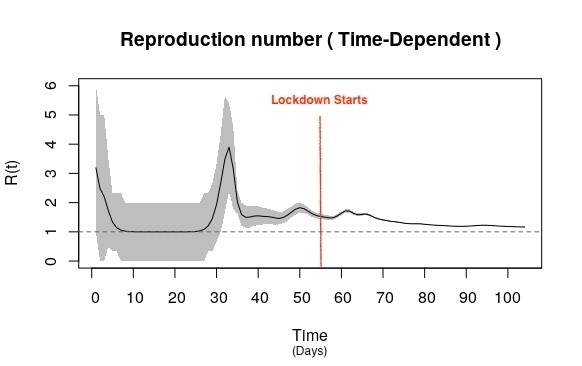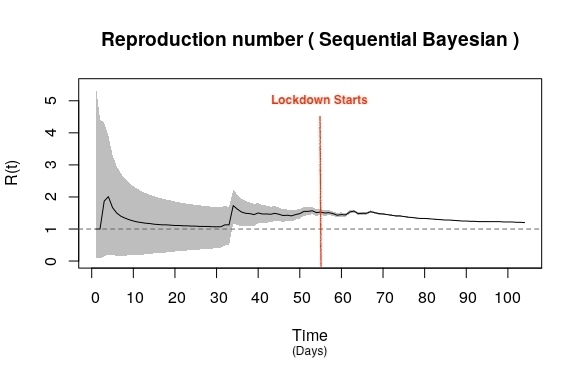# Our COVID-19 Strategy Depends on the R0 Number. Do We Know the R0 Number?The basic reproductive number was until recently to be found inside epidemiology journals and textbooks. But in the last few months, it has become ubiquitous. This number, denoted R0 (pronounced ‘R-zero’), is the average or expected number of people that an infected person could spread the virus to in her ‘infected’ period.

Now, there is reason to believe our strategies to contain the virus’s spread could be founded on mistaken estimates of R0, which then imperils the strategies themselves.

In their 1992 book, Roy Anderson and Robert May wrote, “If R0 is greater than 1, then the outbreak will lead to an epidemic, and if R0 is less than 1, then the outbreak will become extinct.” If R0 is equal to 1, one infected person will infect exactly one other person, and so the number of infected persons in the population will remain constant over time.

R0 helps us determine when herd immunity could be reached. Specifically, (R0 – 1)/R0 is the fraction of individuals in the population who need to be infected to achieve herd immunity. If R0 is 1.66, for example, 40% of the population needs to be infected to achieve herd immunity.

Though epidemiologists can calculate R0 using contact-tracing data, the most common method is to use cumulative incidence data. Specifically, they use individual-level modelling assumptions to put together equations that predict how many people could be infected at different stages. However, the assumptions are hypothetical and mostly unverified.

The number of secondary infections also varies as the epidemic progresses because fewer people remain susceptible to the virus 1, as well as with public-health interventions like lockdowns. So R0 also changes over time. For the purpose of their work, epidemiologists often calculate the R0 at a certain time, denoted R(t).

In either method of estimation, researchers use the number of people who test positive on successive days. Now, the novel coronavirus has been hard to contain because it spreads easily and, relevant to this analysis, it incubates over a 2-14-day period and most of those infected are also asymptomatic. So if there aren’t enough test kits, public health officials could easily miss many of those who have the infection and are potentially spread it to others.

Does the fraction of asymptomatic patients increase uniformly? According to one survey conducted in Sweden, about 2.5% of Stockholmers had an active COVID-19 infection by end-March, which is 30-times the number of people who had tested positive by then. However, within the next four weeks, the number of active cases had ballooned to 100-times those tested. On April 22, Sweden’s chief epidemiologist declared that about 20% of Stockholmers had become immune to the virus. Five days later, it was 30%, according to Sweden’s ambassador to the US. Evidently, the population of asymptomatic patients can increase with time. Caveat: Sweden didn’t impose a lockdown.

Consider the number of cases in India between January 30 (when Kerala reported the first confirmed case) and May 13. The first 55 days were pre-lockdown and the last 50, under the lockdown. Using the data-analysis software R2, I plotted successive R(t) values over time (fig. 1) and a sequential Bayesian model (fig. 2)3.The R(t) was clearly erratic before lockdown but began to dip downward after.

In both plots, the R(t) values peak at around t = 33 – i.e. 33 days after January 30. The lockdown was imposed at t = 55. R(May 13) is close to 1.2 by both the methods. The maximum likelihood estimate of a common value of R0 before lockdown is 1.66, and that for the first 50 days of the lockdown is 1.226.

In India, there is no day-to-day estimate of the number of  asymptomatic COVID-19 patients. So five possibilities arise:

i. The number of asymptomatic cases, which aren’t seen in the data, is a common multiple, such as 2x or 3.5x, of the number of cases detected on that day. If so, the R(t) values will vary exactly as plotted in figures 1 or 2, irrespective of the multiplier.

ii. The number of asymptomatic cases is constant, say 1,000, on each day. The R(t) values in this case will vary thus:

iii. On the first day, there are 180 asymptomatic cases. A 100 new asymptomatic cases are added every successive day such that, on the 105th day, there are 10,580 cases. The R(t) values in this case will vary thus:

iv. On the first day, there are 180 asymptomatic cases. A 100 new asymptomatic cases are added every successive day such that, on the 55th day, there are 5,580 asymptomatic cases. Then, from the 56th day to the 105th, 2,000 new asymptomatic cases are added every day. If so, the R(t) values will vary thus:

v. On the first day, there are 180 asymptomatic cases. A 100 new cases are added every successive day until the lockdown is imposed. Then, for the next 50 days, there are 100 fewer asymptomatic cases each successive day. If so, the R(t) values will vary thus:

(Note that in the final case, R(t) dips below 1 before May 13 where other R(t) models predict an R0 > 1 on this date.)

What is actually happening could of course be very different from what the five possibilities predict. But it seems quite likely that all estimated R(t) values around the world – not just in India – have a problem because almost none of their models accounts for the prevalence of asymptomatic cases.

So before we chalk out strategies to contain the spread of the virus, we must zero in on a common set of assumptions about the prevalence and incidence of asymptomatic cases at different points of time. We could do this through a surveillance programme using serological (i.e. antibody-based) tests. And once we have a more accurate picture, we can develop more effective strategies.

Atanu Biswas is a professor of statistics at the Indian Statistical Institute, Kolkata.

1. Assuming that people who have recovered can’t be reinfected by the same virus

2. The name is a coincidence!

3. A model that accounts for all the data available until that moment instead of just the data at that moment.

Scroll To Top